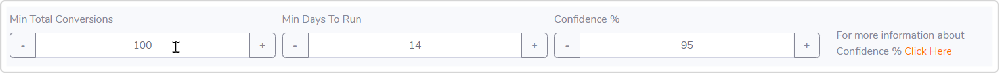# Split Test Settings for Auto-Optimizer

## Split Test Settings for Auto-Optimizer

The Auto-Optimizer test will pick a winner based on your parametersMin Total Conversions – Ideally 100

Reasoning: Anything less is too small a sample to give confidence that the winning result called is actually accurate.

Min Days – Minimum 7 (ideally 14/21 or more in multiples of 7 days)

Reasoning: You need to rule out seasonality and test for full weeks. Eg if you start a test on Monday then you need to end it on a Monday as well. Why? Because conversion rates can vary greatly depending on the day of the week or time of the year (Holidays/Christmas/January Sales etc.)

Confidence % This is the confidence level that the conversion rate measured for the winning variant is accurate – Typically you should aim for a value at or above 95%

#### Confidence Auto Optimizer Test

The system runs a complex algorithm to determine if the confidence level has been reached or not. This confidence algorithm for a particular test is run (and a winner declared if required) when a user either hits a test or hits a conversion.

It may show in stats that all fields are “passed” but a winner won’t yet be chosen.

This will happen the next time either a rotating URL is hit (or a rotating element is displayed) or the conversion is triggered by someone landing on a conversion page (who is already cookied for a rotation page/element).

So… in artificial testing conditions where you might be sending 4 or 5 hits you may see a situation arise where the “winner” is not declared when you expect it to have done so. In a real world scenario with actual traffic hitting these links it will never be an issue and will not, for all practical purposes, happen.

#### Algorithm

To actually understand this better and the algorithm behind the calculation here is the long (and yes complicated explanation – feel free to read it a few times!)

Mathematically, the conversion rate is represented by a binomial random variable, which is a fancy way of saying that it can have two possible values: conversion or non-conversion.

Let’s call this variable as p. Our job is to estimate the value of p and for that we do n trials (or observe n visits to the website). After observing those n visits, we calculate how many visits resulted in a conversion.

That percentage value (which we represent from 0 to 1 instead of 0% to 100%) is the conversion rate of your website.

Now imagine that you repeat this experiment multiple times. It is very likely that, due to chance, every single time you will calculate a different value of p. Having all (different) values of p, you get a range for the conversion rate (which is what we want for next step of analysis).

To avoid doing repeated experiments, statistics has a neat trick in its toolbox.

There is a concept called standard error, which tells how much deviation from average conversion rate (p) can be expected if this experiment is repeated multiple times.

The smaller the deviation, the more confident you can be about estimating the true conversion rate. For a given conversion rate (p) and number of trials (n), standard error is calculated as:

Standard Error (SE) = Square root of (p * (1-p) / n)

Without going much into details, to get 95% range for conversion rate multiply the standard error value by 2 (or 1.96 to be precise). In other words, you can be sure with 95% confidence that your true conversion rate lies within this range: p % ± 2 * SE

#### What Does it Have to do With Reliability of Results?

In addition to calculating conversion rate of the website, we also calculate a range for its variations in an A/B split test.

Because we have already established (with 95% confidence) that true conversion rate lies within that range, all we have to observe now is the overlap between conversion rate range of the website (control) and its variation.

If there is no overlap, the variation is definitely better (or worse if variation has lower conversion rate) than the control. It is that simple.